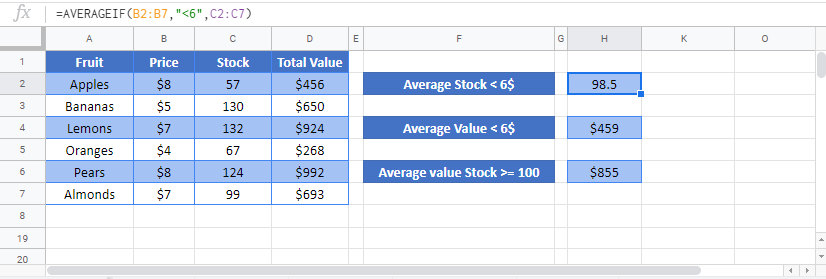# AVERAGEIF & AVERAGEIFS Functions – Average Values If – Excel & Google Sheets

This Tutorial demonstrates how to use the Excel AVERAGEIF and AVERAGEIFS Functions in Excel and Google Sheets to average data that meet certain criteria.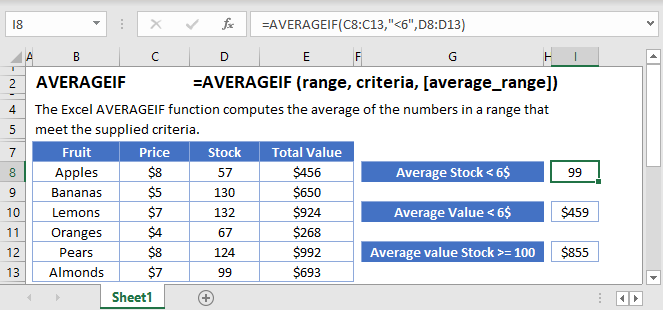## AVERAGEIF Function Overview

You can use the AVERAGEIF function in Excel to count cells that contain a specific value, count cells that are greater than or equal to a value, etc.

To use the AVERAGEIF Excel Worksheet Function, select a cell and type: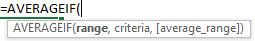(Notice how the formula inputs appear)

## AVERAGEIF Function Syntax and Arguments:

``=AVERAGEIF(range, criteria, [average_range])``

range – The range of cells to count.

criteria – The criteria that controls which cells should be counted.

average_range – [optional] The cells to average. When omitted, range is used.

## What is the AVERAGEIF function?

The AVERAGEIF function is one of the older functions used in spreadsheets. It is used to scan through a range of cells checking for a specific criterion, and then giving the average (aka the mathematical mean) if the values in a range that correspond to those values. The original AVERAGEIF function was limited to just one criterion. After 2007, the AVERAGEIFS function was created which allows a multitude of criteria. Most of the general use remains the same between the two, but there are some critical differences in the syntax that we’ll discuss throughout this article.

If you haven’t already, you can review much of the similar structure and examples in the COUNTIFS article <insert link>.

### Basic example

Let’s consider this list of recorded sales, and we want to know the average income.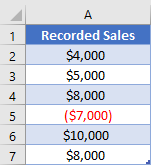Because we had an expense, the negative value, we can’t just do a basic average. Instead, we want to average only the values that are greater than 0. The “greater than 0” is what will be our criteria in an AVERAGEIF function. Our formula to state this is

``=AVERAGEIF(A2:A7, ">0")``

### Two-column example

While the original AVERAGEIF function was designed to let you apply a criterion to the range of numbers you want to sum, much of the time you’ll need to apply one or more criteria to other columns. Let’s consider this table: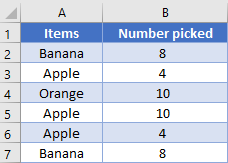Now, if we use the original AVERAGEIF function to find out how many Bananas we have on average. We’ll put our criteria in cell D1, and we’ll need to give the range we want to average as the last argument, and so our formula would be

``=AVERAGEIF(A2:A7, D1, B2:B7)``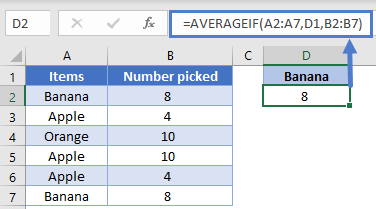However, when programmers eventually realized that users wanted to give more than one criterion, the AVERAGEIFS function was created. In order to create one structure that would work for any number of criteria, the AVERAGEIFS requires that the sum range is listed first. In our example, this means that the formula needs to be

``=AVERAGEIFS(B2:B7, A2:A7, D1)``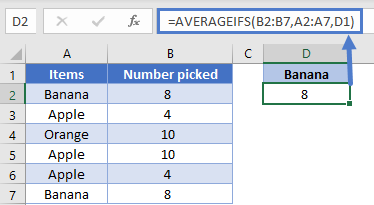NOTE: These two formulas get the same result and can look similar, so pay close attention to which function is being used to make sure you list out all the arguments in the correct order.

### Easy Excel Automation### Working with Dates, Multiple criteria

When working with dates in a spreadsheet, while it is possible to input the date directly into the formula, it’s best practice to have the date in a cell so that you can just reference the cell in a formula. For example, this helps the computer know that you’re wanting to use the date 5/27/2020, and not the number 5 divided by 27 divided by 2020.

Let’s look at our next table recording the number of visitors to a site every two weeks.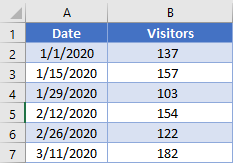We can specify the start and end points of the range we want to look at in D2 and E2. Our formula then to find the average the number of visitors in this range could be:

``=AVERAGEIFS(B2:B7, A2:A7, ">="&D2, A2:A7, "<="&E2)``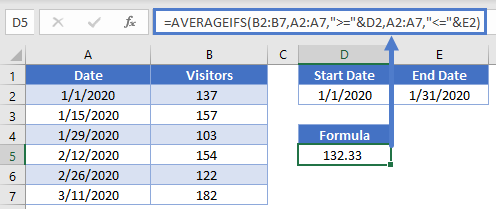Note how we were able to concatenate the comparisons of “<=” and “>=” to the cell references to create the criteria. Also, even though both criteria were being applied to the same range of cells (A2:A7), you need to write out the range twice, once per each criterion.

### Multiple columns

When using multiple criteria, you can apply them to the same range as we did with previous example, or you can apply them to different ranges. Let’s combine our sample data into this table: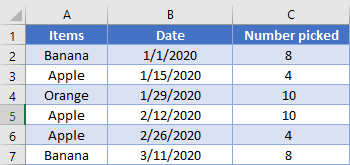We’ve setup some cells for the user to enter what they want to search for in cells E2 through G2. We thus need a formula that will add up the total number of apples picked in February. Our formula looks like this:

``=AVERAGEIFS(C2:C7, B2:B7, ">="&F2, B2:B7, "<="&G2, A2:A7, E2)``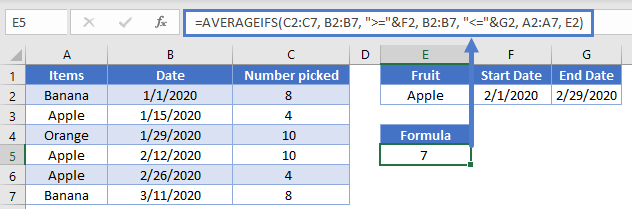### AVERAGEIFS with OR type logic

Up to this point, the examples we’ve used have all been AND based comparison, where we are looking for rows that meet all our criteria. Now, we’ll consider the case when you want to search for the possibility of a row meeting one or another criterion.

Let’s look at this list of sales: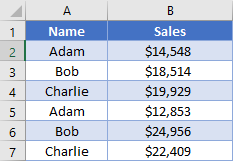We’d like to add up the average sales for both Adam and Bob. First, a quick discussion about taking averages. If you have an uneven number of things such has 3 entries for Adam and 2 for Bob, you can’t simply take the average of each person’s sales. This is known as taking the average of averages, and you end up giving an unfair weighting to the item that has few entries. If this is the case with your data, you’d need to calculate an average the “manual” way: take the sum of all your items divided by the count of your items. To review how to do this, you can check out the articles here: <link to SUMIFS and COUNTIFS>

Now, if the number of entries is the same, such as in our table, then you have a couple options you can do. The simplest is to add two AVERAGEIFS together, like so, and then divide by 2 (the number of items in our list)

``=(AVERAGEIFS(B2:B7, A2:A7, "Adam")+AVERAGEIFS(B2:B7, A2:A7, "Bob"))/2``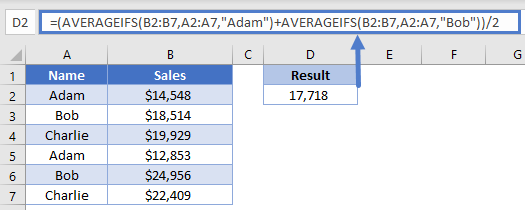Here, we’ve had the computer calculate our individual scores, and then we add them together.

Our next option is good for when you have more criteria ranges, such that you don’t want to have to rewrite the whole formula repeatedly. In the previous formula, we manually told the computer to add two different AVERAGEIFS together. However, you can also do this by writing your criteria inside an array, like this:

``=AVERAGE(AVERAGEIFS(B2:B7, A2:A7, {"Adam", "Bob"}))``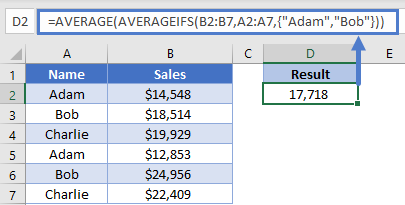Look at how the array is constructed inside the curly brackets. When the computer evaluates this formula, it will know that we want to calculate an AVERAGEIFS function for each item in our array, thus creating an array of numbers. The outer AVERAGE function will then take that array of numbers and turn it into a single number. Stepping through the formula evaluation, it would look like this:

``````=AVERAGE(AVERAGEIFS(B2:B7, A2:A7, {"Adam", "Bob"}))
=AVERAGE(13701, 21735)
=17718``````

We get the same result, but we were able to write out the formula a bit more succinctly.

### Dealing with blanks

Sometimes your data set will have blank cells that you need to either find or avoid. Setting up the criteria for these can be a little tricky, so let’s look at another example.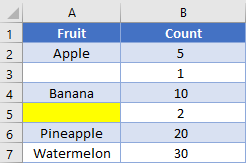Note that cell A3 is truly blank, while cell A5 has a formula returning a zero-length string of “”. If we want to find the total average of truly blank cells, we’d use a criterion of “=”, and our formula would look like this:

``=AVERAGEIFS(B2:B7,A2:A7,"=")``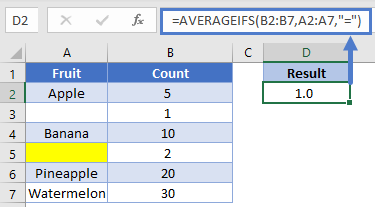On the other hand, if we want to get the average for all cells that visually looks blank, we’ll change the criteria to be “”, and the formula looks like

``=AVERAGEIFS(B2:B7,A2:A7,"")``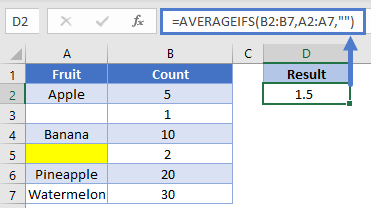Let’s flip it around: what if you want to find the average of non-blank cells? Unfortunately, the current design won’t let you avoid the zero-length string. You can use a criterion of “<>”, but as you can see in the example, it still includes the value from row 5.

``=AVERAGEIFS(B2:B7,A2:A7,"<>")``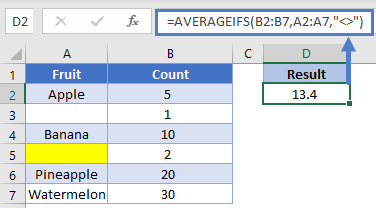If you need to not count cells containing zero length strings, you’ll want to consider using the LEN function inside a SUMPRODUCT <link to SUMPRODUCT article).